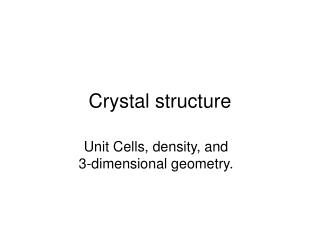DownloadDownload PresentationCrystal structure

# Crystal structure

Download Presentation## Crystal structure

- - - - - - - - - - - - - - - - - - - - - - - - - - - E N D - - - - - - - - - - - - - - - - - - - - - - - - - - -
##### Presentation Transcript

1. Crystal structure Unit Cells, density, and 3-dimensional geometry.

2. Cubic crystal structures • Simple cubic (sc) • Body-centered cubic (bcc) • Face-centered cubic (fcc)

3. How many atoms/cell? • A corner atom has 1/8 atom inside the cube. • A body-centered atom is entirely within the cube • A face-centered atom has ½ atom inside the cube

4. Cubic crystal structures SC BCC FCC SC (8 x 1/8)=1 atom/cell BCC (8 x 1/8)+1=2 atoms/cell FCC (8 x 1/8)+ (6 x ½)=4 atoms/cell

5. a=edge of a cell, r=radius of atom • SC a=2r

6. a=edge of a cell, r=radius of atom • BCC (√3)a=4r

7. a=edge of a cell, r=radius of atom • FCC (√2)a=4r

8. A word about volume • r is usually expressed in picometers(10-12m) • V (cube)= a3 • Your best bet is to convert: mm3Lml

9. Conversion factors • L=6.02 x 1023 atoms/mol • D=__g/ml • FM=___g/mol • V=___ml/cell • 1m3=1000L • 1L=1000ml

10. Tasks • What is the crystal structure? • What is the radius of the atom? • What is the volume of the unit cell? • What is the density?

11. Tasks • What is the crystal structure? Find atoms/cell • What is the radius of the atom? Find r • What is the volume of the unit cell? Find a3 • What is the density? Find g/ml

12. Ex: • What is the crystal structure? Find atoms/cell Manganese has a density of 7.4 g/ml, the length of the unit cell is 291 pm.

13. Ex: • What is the radius of the atom? Find r Chromium has a BCC structure and a density of 7.19 g/ml

14. Ex: • What is the volume of the unit cell? Find a3 Copper has a FCC structure and a density of 8.96 g/ml

15. Ex: • What is the density? Find g/ml Strontium has BCC structure and r=215 pm

16. Ex: • What is the crystal structure? Find atoms/cell Nickel has a density of 8.9 g/ml. Its atomic radius is 125 pm